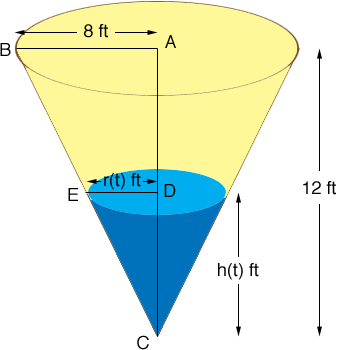SEARCH HOMEMath Central Quandaries & QueriesQuestion from mike, a student: A water tank has the shape of a right circular cone with height 12 feet and radius 8 feet. Water is running into the tank so that the radius r (in feet) of the surface of the water is given by r=0.75t where t is the time (in minutes) that the water has been running. the volume V of the water is given by V=1/3 pi r^2h. Find V(t) and use it to determine the volume of the water when t=5 minutes.Hi Mike,

What you are missing is the height, h(t) of the water at time t minutes. Similar triangles helps determine this.Triangles ABC and DEC are similar so

12/8 = h(t)/r(t).

You know that r(t) = 0.75 ft so substitute into this expression and solve for h(t). Now substitute r(t) and h(t) into the expression for the volume to determine Vt).

PennyMath Central is supported by the University of Regina and The Pacific Institute for the Mathematical Sciences.